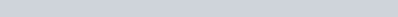In this tutorial I look at the locus of a point which is equidistant from two fixed points A and B in the complex plane by considering the following equation.

Equation of a perpendicular bisector has the form:

•You are given an example to try during the video with the usual worked solution.

Complex Numbers - Loci : Perpendicular bisector : ExamSolutions Maths Video Tutorials - youtube Video